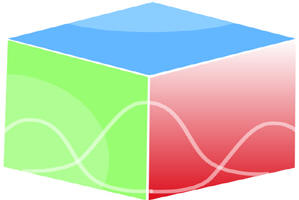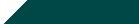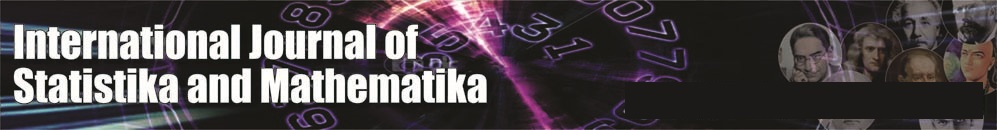``Home| Journals | Statistics Online Expert | About Us | Contact UsUntitled Document

Laplace - Modified Decomposition Method to Study Solitary Wave Solutions of Coupled Nonlinear Klein-Gordon Schrödinger Equation

Ram Dayal Pankaj

Department of Mathematics and Statistics, J. N. V. University, Jodhpur, Rajasthan, INDIA.

[email protected]

Research Article

Abstract: Analytical and numerical solutions are obtained for coupled Klein-Gordon Schrödinger Equation by the well-known Laplace Modified decomposition method [LMDM]. We combined Laplace transform and Modified decomposition method and present a new approach for solving Coupled Klein-Gordon Schrödinger equation. The method does not need linearization, weak nonlinearity assumptions, or perturbation theory. We compared the numerical solutions with corresponding analytical solutions.

Keywords: Laplace Modified decomposition method, Laplace transforms, Coupled Klein-Gordon Schrödinger Equation.

1. Introduction

Systems of partial differential equations have attracted much attention in a variety of applied sciences. The general ideas and the essential features of these systems are of wide applicability. These systems were formally derived to describe wave propagation , to control the shallow water waves , and to examine the chemical reaction-diffusion model of Brusselator . The method of characteristics, the Riemann invariants, and Adomian method  were the commonly used methods.

In this paper; we shall consider the coupled nonlinear Klein-Gordon Schrödinger equations in the form

(1)

The modified decomposition method has been applied for solving coupled Klein-Gordon- Schrödinger equations which play an important role in modern physics. It describes a system of conserved scalar nucleons interacting with neutral scalar mesons coupled through the Yukawa interaction [19 20 21]. Fan et al.  have been proposed an algebraic method to obtain the explicit exact solutions for coupled Klein-Gordon-Schrödinger (K-G-S) equations. Recently, The Jacobi elliptic function expansion method has been applied to obtain the solitary wave solutions for coupled K-G-S equations .   The ADM, which accurately computes the series solution, is of great interest to applied sciences [16-18]. The method provides the solution in a rapidly convergent series with components that can be elegantly computed. The nonlinear equations are solved easily and elegantly without linearizing the problem by using the ADM [16-17]. Recently, the solution of fractional differential equation has been obtained through Adomian decomposition method by the researchers [22-24]. The method has features in common with many other methods, but it is distinctly different on close examinations, and one should not be mislead by apparent simplicity into superficial conclusions   [16, 17]. In this work, we used Laplace modified decomposition method introduced by Khuri [7, 8] which is further used by Yusufoglu to solve Duffing equation  and Elgasery for Falkner- Skan equations  Schrödinger -KdV (Sch-KdV) equation. This technique, modified by Hussain and Khan , illustrates how the Laplace transform may be used to approximate the solutions of the nonlinear partial differential equations by extending the decomposition method [12, 13].

1. Analysis of the Method

We consider the coupled non linear K-G-S equations (1) in the form

(2)

Where , is considered a first and second order partial differential operator,  is linear and   are symbolize nonlinear operators respectively, The method consists of first applying the Laplace transform to both sides of equations in system (2) with differentiation property of Laplace transform and taking inverse Laplace transform ,we get

(3)

The Adomain decomposition method [16, 17] assumes infinite series solutions for unknown function E(x, t) and η(x, t) by the infinite series given by

(4)

The nonlinear terms   are usually represented by an infinite series of the so-called Adomian polynomials 

(5)

The Adomian polynomials can be generated for all forms of nonlinearity. They are determined by the following relations:

(6)

Similarly

(7)

This formula is easy to set computer code to get as many polynomials as we need in calculation of the numerical as well as explicit solutions. For the sake of convenience of the readers, we can give the first few Adomian polynomials for   of the nonlinearity as

And

and so on, the rest of the polynomials can be constructed in a similar manner. Substituting the initial conditions into (3) identifying the zeroth components and then we obtain the subsequent components by the following recursive equations by using the standard ADM

(8)

Recently, Wazwaz  proposed that the construction of the zeroth component of the decomposition series can be defined in a slightly different way. In , he assumed that if the zeroth component  and the function g is possible to divide into two parts such as and  , the one can formulate the recursive algorithm for  and general term in a form of the modified  recursive scheme as follows:

(9)

Similarly, if the zeroth component  and the function is possible to divide into two parts such as and

(10)

This type of modification is giving more flexibility to the ADM in order to solve complicate nonlinear differential equations. In many cases the modified decomposition scheme avoids the unnecessary computation especially in calculation of the Adomian polynomials. The computation of these polynomials will be reduced very considerably by using the MDM.

It is worth noting that the zeroth components and are defined then the remaining component and , can be completely determined. As a result, the components  and areidentified and the series solutions thus entirely determined. However, in many cases the exact solution in aclosed form may be obtained.

The decomposition series (4) solutions are generally converges very rapidly in real physical problems .The rapidity of this convergence means that few terms are required. Convergence of this method has been rigorously established by Cherruault , Abbaoui and Cherruault [27, 28] and Himoun, Abbaoui and Cherruault . The practical solutions will be the n -term approximations and

(11)

With

1. Implementation of the method

We first consider the application of coupled K-G-S equations (1) with the initial conditions

(12)

Where rand k are arbitrary constants

Using (9) and (10) with (6) and (7) respectively and considering  for the coupled K-G-S equations (1) and initial conditions (12) gives

................................................................

and

...............................................................................

and so on, in this manner the other components of the decomposition series can be easily obtained of which E(x, t) and η(x, t) were evaluated in a series form

...........  (13)

.............................................. (14)

1. Numerical Results and Discussions

In the present numerical experiment eq. (13) and (14) have been used to draw the graphs as shown in Figs.1, 2 respectively. The numerical solutions of the coupled K-G-S equations (1) have been shown in Fig. 1 Fig. 2 with the help of five-term and four-term approximations  and for the decomposition series solutions of E(x, t) and η(x, t) respectively. In the present numerical computation we have assumed r = 0.05 and k = 0.05

(a)                                                                               (b)

Figure 1: (a) The decomposition method solution for Abs (E(x, t)), (b) Corresponding solution for Abs (E(x, t)) when t = 0

(a)                                                                                (b)

Figure2: (a) The decomposition method solution for η(x, t), (b) Corresponding solution for η(x, t) when t = 0

1. Conclusion

The Laplace Modified decomposition method [LMDM] is a powerful method which has provided an efficient potential for the solution of coupled Klein-Gordon Schrödinger Equation with initial condition. The approximate solutions to the equations have been calculated by using the method [LMDM] without any need to a transformation techniques and linearization of the equations. Additionally, it does not need any discretization method to get numerical solutions. This method thus eliminates the difficulties and massive computation work. The algorithm can be used without any need to complex calculations except for simple and elementary operations.

References

1. L. Debnath, Nonlinear Partial Differential Equations for Scientists and Engineers, Birkhauser,Boston, 1997.
2. J.D. Logan, an Introduction to Nonlinear Partial Differential Equations, Wiley-Interscience, New York, 1994.
3. G.B. Whitham, Linear and Nonlinear Waves, Wiley, New York, 1974.
4. C. Lubich, A. Ostermann, Multigrid dynamic interaction for parabolic equations, BIT 27(1987) 216 - 234.
5. S. Vandewalle, R. Piessens, Numerical experiments with nonlinear multigrid wave form relaxation on a parallel processor, Appl. Numer. Math. 8 (1991) 149 - 161.
6. G.Adomian,The diffusion-Brusselator equation,Comput. Math. Appl.29 (1995) 1-3.
7. S.A. Khuri, A Laplace decomposition algorithm applied to class of nonlinear differential equations, J Math. Appl. 4 (2001) 141 - 155.
8. S. A.Khuri, A new approach to Bratu’s problem, Appl.Math.Comput. 147 (2004)131-136.
9. Elcin Yusufoglu (Agadjanov), Numerical solution of Duffing equation by Laplace decomposition algorithm, Appl. Math. Comput. 177 (2006) 572 - 580.
10. S. Nasser Elgazery, Numerical solution for the Falkner-Skan equation, Chaos, Solitons and Fractals, 35 (2008) 738 - 746.
11. A.M. Wazwaz, Partial differential equations methods and applications, Netherland Balkema Publisher, 2002.
12.  Arun Kumar and R.D. Pankaj Laplace DecompositionMethod to Study SolitaryWave Solutions of Coupled Nonlinear Partial Differential Equation Computational MathematicsVolume 2012, Article ID 423469, 5 pages.
13. M. Hussain and M. Khan, Modified Laplace decomposition method, Appl. Math. Sci. 4 (36) (2010)1769-1783
14. Darwish, A. and Fan, E. G.: A series of New Explicit Exact Solutions for the Coupled Klein - Gordon- Schrödinger Equations. Chaos Soliton & Fractals. 20,609-617(2004)
15.  Liu, S., Fu, Z., Liu, S., and Wang, Z.: The Periodic Solutions for a Class of Coupled Nonlinear Klein-Gordon Equations. Physics Letters A. 323,415-420(2004)
16. Adomian G.: Nonlinear Stochastic Systems theory and Applications to Physics. Kluwer Academic publishers Netherlands(1989)
17. Adomian G.: Solving frontier problems of physics: The decomposition method. Kluwer Academic Publishers. Boston(1994)
18. Wazwaz, A. M.: Partial Differential Equations: Methods and Applications. Balkema, Rotterdam.(2002)
19. H. Added and S. Added, Equations of Langmuir turbulence and nonlinear Schrödinger equation: Smoothness and approximation, J. Funct. Anal., 79 (1988), pp. 183–210.
20. I. Fukuda and M. Tsutsumi, On the Yukawa-coupled Klein-Gordon-Schrödinger equations in three space dimensions, Prod. Japan Acad., 51 (1975), pp. 402–405.
21. V. G.Makhankov,Dynamics of classical solitons(in non integrable systems), Phys. Rep., 35 (1978), pp. 1–128.
22. Saha Ray, S. Bera, R. K.:Analytical Solution of a Dynamic System Containing Fractional Derivative of Order one-half by Adomian Decomposition Method . Transaction ASME Journal of Applied Mechanics. 72(2), 290-295(2005)
23. Saha Ray, S. Bera, R. K. : An Approximate Solution of a Nonlinear Fractional Differential Equation by Adomian Decomposition Method . Applied Mathematics and Computation. 167(1), 561-571(2005)
24. Saha Ray, S. Bera, R. K.: Analytical Solution of the Bagley Torvik Equation by Adomian Decomposition Method. Applied Mathematics and Computation. 168(1), 398-410(2005)
25. Wazwaz, A. M.: A Reliable Modification of Adomian Decomposition Method . Appl. Math. Comp...102(1), 77-86(1999)
26. Cherruault, Y.: Convergence of Adomian’s Method. Kybernetes . 18, 31-38(1989)
27. Abbaoui, K. Cherruault, Y.: Convergence of Adomian’s Method Applied to Differential Equations .Computers Math. Applic.. 28 (5), 103-109(1994)
28. Abbaoui, K. Cherruault, Y.: New Ideas for Proving Convergence of Decomposition Methods  Computers Math. Applic. . 29, 103-108(1995)
29. Himoun, N., Abbaoui, K. Cherruault, Y.: New Results of Convergence of Adomia.